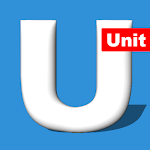ID: calculation.apps.handyunitsconverter

The description of Unit Converter: Feet to Meter

Handy Unit Converter app is a simple and an amazing unit conversion tool, which is developed keeping in mind everyday use of the unit calculator in different tasks throughout the day. It is a universal unit converter calculator app which is capable of converting units of different categories. Simple to use with an amazing and user-friendly interface.

Handy Unit Converter is a calculator App to calculate all common and basic units conversion including common units conversion, Electrical Engineering units conversion, civil engineering units conversion and Math tools.

Common unit converters contain the following unit conversion:
• Length unit converter.
• Area unit converter.
• weight unit converter.
• Volume unit converter.
• Time unit converter.
• Speed unit converter.
• Voltage unit converter.
• Current unit converter.
• Resistance unit converter.
•Temperature unit converter.
• Pressure unit converter.
• power unit converter.
• Force unit converter.
• Work unit converter.
• Fuel unit converter.
• Data unit converter.
• Angle unit converter
• Energy unit converter

Electrical/Electricity unit converter contains the following unit conversion:
• charge unit converter.
• Linear charge density converter.
• Surface charge density converter.
• Volume charge density converter.
• Electric potential unit converter.
• Electric field strength unit converter.
• Capacitance Calculator.
• inductance converter.
• Electrical conductivity.
• Magnetic flux converter.
• Magnetic flux density.
• Conductance converter.
• Moment of inertia.
• Acceleration converter.

Civil engineering unit converters contain the following unit conversion:
• Flow unit converter.
• Flow mass converter.
• Material weight converter.
• Round steel weight.
• Dynamics viscosity.
• Kinematics viscosity.
• Surface tension unit converter.

Math tool contains the following Calculator:
• Direct proportion calculator.
• Inverse proportion calculator.
• Statistics calculator.
• Circle area calculator.
• Rectangle area calculator.
• Triangle area calculator.
• Cone volume calculator.
• Cylinder volume calculator.
• Cube volume calculator.
• perimeter of square, rectangle and triangle calculator.
• Surface area of cube calculator.
• Surface area of sphere calculator.
• Surface area of cylinder calculator.
• Linear equation calculator.
• Ratio calculator.